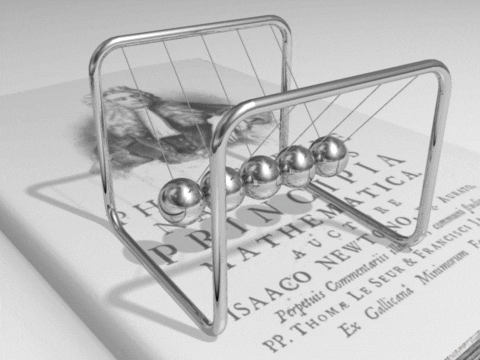# CPA Physics

• Welcome to the CPA Physics.

We discover various phenomena found in nature and understand why they occur.

Physics is the study of matter, energy, and their interactions.  In Physics we cover the following topics:

• Linear Motion
• Projectile Motion
• Forces in 1 Dimension
• Forces in 2 Dimensions
• Uniform Circular Motion and Gravitational Forces
• Momentum
• Work and Energy
• Static Electricity
• Current Electricity
• Magnetism
• Waves
• Sound
• Light

This is a full year college preparatory level course in introductory physics. At the conclusion of the course, students will be able to understand and explain physical events and conditions that students see regularly in their lives. To help students learn, we will use a combination of classroom discussions, calculation practice, and lab experiments.  Students will be asked to think creatively, actively participate in class, understand mathematical relationships and calculations, and to work individually and in small groups. I hope students find this course enjoyable and reference theis class experience and education when deciding on future studies.